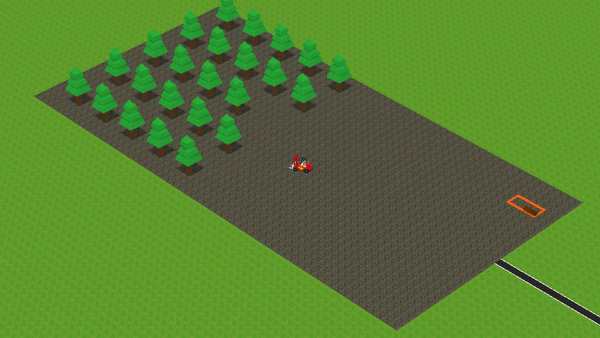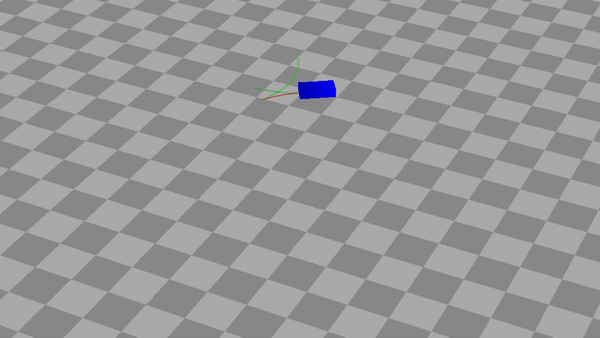# RSMotion - C++ Library for Reeds-Shepp Cars

For calculating optimal car motions in open spaces. Useful for games, robotics and simulations.In a logistics game I have been working on there are trucks picking up goods and delivering then. The game needs to calculate their paths in order to simulate their motions. On normal roads the trucks simply follow a determined path as defined by the road lanes, but I needed a different approach when the trucks are driving across an open space such as a big parking lot or when trying to manoeuvre towards a free terminal. This is where Reeds-Shepp cars come in! This algorithm will find the optimal path using turns, straights and even by going in reverse. This allows the truck to navigate efficiently and convincingly in open spaces.Example of in-game usage of the RSMotion library. The forklift truck is picking up trees and bringing them to storage. The turns and straights are defined by Reeds-Shepp paths.

I have extracted the functionality into a library as it might be useful for other developers as well. The library is named RSMotion and is now available on GitHub. It is based on code in OPML but adapted and rewritten to strip dependencies and to provide a more modern C++ interface.

Curious about the algorithm? Below is a short introduction.

# Calculating paths for cars

Let’s assume a car has the following freedoms: 1) going forward or in reverse and 2) turning left or right. What is the optimal path for the car to go from point A to point B?

The library calculates this optimal path by using Reeds-Shepp Cars. Based on a start position and destination it provides the path segments that define the optimal path. Each path segment is defined by its type (a turn or straight), length and direction (forward or reverse).

In the video below you can see what the generated paths look like.Example of Reeds-Shepp paths. The car goes forwards on green path segments, and in reverse on red segments.

## How does it work?

Well, the best way to really know how it works, is to read the paper by Reeds and Shepp. The calculations and proofs are quite involved but fortunately we only have to implement it.

Basically, each path is defined by three to five path segments. Each segment can be either a turn (notated as the character `C`) or a straight (notated as the character `S`). For example, a path with 1) a turn, 2) a straight, and 3) a turn can be notated as the word `CSC`. Another example would be three turns: `CCC`. Note that a turn can either go left or right. Also, a turn can be a of 0 degrees which essentially reduces the turn to a no-op.

As it turns out, all optimal paths can be defined by one of five words. This is reflected in the code:

``````Path SearchShortestPath(State toState)
{
// the optimal path is always one of
// these five path configurations
return std::min({CSC(toState),
CCC(toState),
CCCC(toState),
CCSC(toState),
CCSCC(toState)});
}
``````

For each of these five configurations we test different variants of these paths by reflecting and time-flipping (going in reverse) them. Then, out of all valid paths (the paths that actually reach the destination) we take the path with the minimum length:

``````
template <class R>
Path CalculateMinPath(Path basePath, State toState, R baseFormula)
{
Path minPath;

// for each equation, try each operation and return the shortest
if (baseFormula(toState, basePath))
{
minPath = std::min(minPath, basePath);
}
if (baseFormula(Reflect(toState), basePath))
{
minPath = std::min(minPath, Reflect(basePath));
}
if (baseFormula(Timeflip(toState), basePath))
{
minPath = std::min(minPath, Timeflip(basePath));
}
if (baseFormula(Reflect(Timeflip(toState)), basePath))
{
minPath = std::min(minPath, Reflect(Timeflip(basePath)));
}

return minPath;
}
``````

As you can see the algorithm is a kind of brute-force approach in that it calculates a lot of alternatives and simply picks the one with the smallest length. However, the result is proven to be optimal and the number of paths to test is always the same. I have not done any measurements on the runtime but I expect it to not vary much between different searches. It certainly is not dependent on the path length.

## Repository

Checkout the library at GitHub.